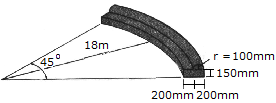# Engineering Mechanics - Center of Gravity and Centroid - Discussion

### Discussion :: Center of Gravity and Centroid - General Questions (Q.No.4)

4.Determine the volume of concrete needed to construct the circular curb.

 [A]. V = 1.083 m3 [B]. V = 1.309 m3 [C]. V = 1.756 m3 [D]. V = 8.67 m3

Explanation:

No answer description available for this question.

 Vanessa said: (Jan 24, 2015) Could anyone please explain how to properly approach this problem using the Pappus-Guldinas theorem.

 Jack said: (Dec 28, 2015) I didn't use the Pappus-Guldinas theorem but I solved this question by using basic mathematical formulas. Firstly, I found the interior side which sees the angle is 45 degree from (45*(2*pi*(18+0.4m))/360. Then with the same way I found the exterior side. And the I calculated the volume of the bottom part. Finally, I calculated the volume of the upper part and addition of two volume and I got 1.073 m^3. I chose the nearest option C.

 John said: (Jan 18, 2016) I solved using Pappus theorem. I got 1.079, I choose A.

 Susheel said: (Feb 29, 2016) Tell me the Pappus theorem.

 Katharnak said: (Jul 12, 2017) I think Sambar approach is better than Pappus.